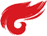java 基础
• (单选题)下列有关实参和形参说法正确的是（A
• A)形参是指在定义方法时参数列表中的参数 实参是指在调用方法时所传入的实际数据
• B)形参变量的作用域是在整个类中都可以使用
• C)实参只能是字面量值不可以是变量
• D)形参不可以用 final 修饰
• 解析：形参是指在定义方法时参数列表中的参数，实参是指在调用方法时所传入的实际数据。 形参是局部变量，作用域在方法体内，出了方法后不可见。 实参可以是字面量常量值也可以是变量，只要是可以在调用方法时传入的数据就是实参。 形参是可以用 final 来修饰的，被 final 修饰的形参被赋初值后值不可以再改变。 所以 A 正确。

• (单选题)编译运行以下程序后，关于输出结果的说明正确的是（C ） publicclass Conditional{ publicstaticvoid main(String args[]){ int x=4; System.out.println("value is "+ ((x>4) ? 99.9 : 9)); } }
• A)输出结果为：value is 99.99
• B)输出结果为：value is 9
• C)输出结果为：value is 9.0
• D)编译错误
• 解析：三目运算符中：第二个表达式和第三个表达式中如果都为基本数据类型，整个表达式的运算结果由容量高的决定。99.9是double类型而9是int类型， double 容量高。

• (单选题)java中char类型的取值范围是（）
• A)0 ... 32767
• B)0 ... 65535
• C)–256 ... 255
• D)–32768 ... 32767

• 正确答案为：B
解析：在java中，char是一个无符号16位类型，取值范围为0到65535

• (单选题)选择下面代码的运行结果：（ ） public class Test{ public void method(){ for(int i = 0; i < 3; i++){ System.out.print(i); } System.out.print(i); } }
• A)0122
• B)0123
• C)编译错误
• D)没有任何输出

• 正确答案为：C
解析：i变量的作用范围是整个for循环中

• (单选题)隐式类型转换是由按优先关系从低到高的顺序，下列描述正确的顺序为（ ）
• A)char-int-long-float-double
• B)int-long-float-double-char
• C)long-float-int-double-char
• D)以上都不对

• 正确答案为：A
解析：隐式类型转换规则是： byte→short(char)→int→long→float→double

• (单选题)阅读下列代码; public class Test{ String s="One World One Dream"; public static void main(String args[]){ System.out.println(s); } } 其运行结果是（）
• A)args
• B)One World One Dream
• C)s
• D)编译错误

• 正确答案为：D
解析：main方法是程序执行的入口，在本程序中main方法中的语句"System.out.println(s);"无法调用在main方法外声明的变量，所以编译时出错。

• (多选题)下列说法错误的有()
• A)数组是一种对象
• B)数组属于一种原生类
• C)int number=[]={31,23,33,43,35,63}
• D)数组的大小可以任意改变

• 正确答案为：BCD
解析：数组不是原生类（即基本数据类型）：int double boolean float byte short long char ，初始化多大就是多大

• (单选题)下列数组的初始化正确的是（）
• A)int[ ] score = new int[ ];
• B)int score[ ] = new int[ ] { 34, 90, 87, 54, 24 };
• C)int[ ] score = new int[ 4 ] { 90, 12, 34, 77 };
• D)int score = { 78, 23, 44, 78 }；

• 正确答案为：B
解析：选项 A 需要指定数组的长度；选项 C 在声明数组的同时赋值时不能指定数组长度；选项 D 声明数组的语法错误，应为int[ ] score={ 78, 23, 44, 78 }；

• (多选题)下列哪些选项属于Java技术体系（）
• A)Java Me
• B)Java Se
• C)Java EE
• D)Java Card

• 正确答案为：ABCD
解析：Java Card、Java Me、Java Se、Java EE都属于Java技术体系

• (多选题)下列选项中创建数组能够编译通过的是（ ）
• A)int[] ia = new int ;
• B)float fa = new float ;
• C)char[] ca = "Some String";
• D)Object oa = new float;

解析：任何类的父类都是 Object，数组属于引用类型，Object oa = new float;这种写法相当于父类的引用指向子类的实例。

• 多选题)byte b1=1,b2=2,b3,b6,b8; final byte b4=4,b5=6,b7; b3=(b1+b2); /*语句1*/ b6=b4+b5; /*语句2*/ b8=(b1+b4); /*语句3*/ b7=(b2+b5); /*语句4*/ System.out.println(b3+b6); 下列代码片段中，存在编辑错误的语句是()
• A)语句2
• B)语句1
• C)语句3
• D)语句4

• 正确答案为：BCD
解析：语句1错误：b3=(b1+b2);自动转为int，所以正确写法为b3=(byte)(b1+b2);或者将b3定义为int； 语句2正确：b6=b4+b5;b4、b5为final类型，不会自动提升，所以和的类型视左边变量类型而定，即b6可以是任意数值类型； 语句3错误：b8=(b1+b4);虽然b4不会自动提升，但b1仍会自动提升，所以结果需要强转，b8=(byte)(b1+b4); 语句4错误：b7=(b2+b5); 同上。同时注意b7是final修饰，即只可赋值一次，便不可再改变。

• (单选题)存储一个24X24点阵的汉字（每个点1bit），需要多少字节（ ）
• A)24
• B)48
• C)72
• D)144

• 正确答案为：C
解析：24*24/8=72。意思是：24*24的结果是二进制位，而八个二进制位等于 一个字节，所以再除以八是所得的字节数。

• (单选题)下面哪个是正确的（ ）
• A)String temp [] = new String {"a""b""c"};
• B)String temp [] = {"a""b""c"};
• C)String temp = {"a", "b", "c"};
• D)String temp [] = {"a", "b", "c"};

• 正确答案为：D
解析：数组声明初始化时前面的接收变量类型应该是数组类型，需要具有"[]"号， 且数组中每个元素都必须是统一指定类型，并且元素间需要使用","号分隔。

• (单选题)以下代码的执行结果是（） public class Test { private static float[] f = new float; public static void main(String args[]) { System.out.println("f = " + f); } }
• A)f = 0
• B)f = 0.0
• C)代码不能通过编译
• D)程序运行时出错

• 正确答案为：B
解析：float型数组中的元素都是float，默认初始值是0.0。



1. abstract class Vehicle {
2.     public int speed() {
3.         return 0;
4.     }
5. }
6.
7. class Car extends Vehicle {
8.     public int speed() {
9.         return 60;
10.     }
11. }
12.
13. class RaceCar extends Car {
14.     public int speed() {
15.         return 150;
16.     }
17. }
18.
19. public class TestCar {
20.     public static void main(String[] args) {
21.         RaceCar racer = new RaceCar();
22.         Car car = new RaceCar();
23.         Vehicle vehicle = new RaceCar();
24.         System.out.println(racer.speed() + ", " + car.speed() + ", "
25.      + vehicle.speed());
26.     }
27. }

A. 0,0,0

B. 150,60,0

C. 150,150,150

D. 抛出运行时异常

C 选项正确。

posted on 2019-03-20 15:16  jeasion  阅读(346)  评论(0编辑  收藏